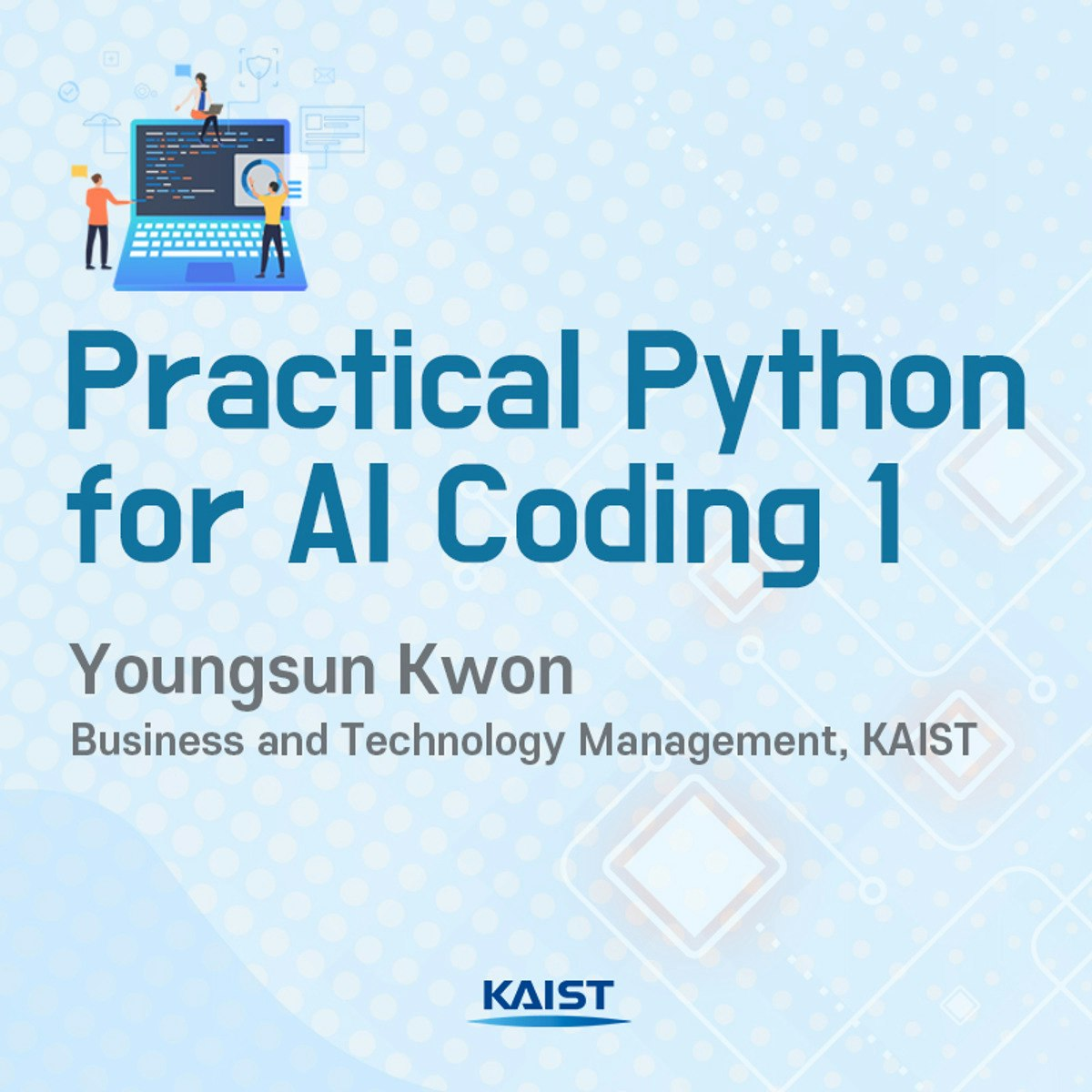# Write a Java Program that find the factorial of a number

Contents

## How to find the factorial of a number in java

In this program, we will learn to write a Java program that finds the factorial of a number.

### Algorithm to find factorial of a number

1. import java.io.*; and import java.util.Scanner; // package
2. Create: class Factorial{
3. Create the main function:
1. public static void main(String args[])
4. create integer variable:
1. int n, c, fact = 1;
5. Print the message to use to enter an integer to calculate it’s factorial
6. Scanner in = newScanner(System.in);
8. if( n < 0){
9. System.out.println(“Number should be non-negative.”);
10. else {

11. for ( c = 1 ; c <= n ; c++ )

12. fact = fact*c;

13. System.out.println(“Factorial of “+n+” is = “+fact);

### Source Code:

`import java.io.*;import java.util.Scanner;class Factorial{public static void main(String args[]){int n, c, fact = 1;System.out.println("Enter an integer to calculate it's factorial:");Scanner in=new Scanner(System.in);n =in.nextInt();if ( n < 0 )System.out.println("Number should be non-negative.");else{for(c= 1 ; c <=n ; c++)fact =fact*c;System.out.println("Factorial of "+n+" is ="+fact);}}}`

### Expected Output:

Enter an integer to calculate it’s factorial : 6
Factorial of 6 is = 720## FAQ:

### Is there a factorial function in Java?

No there is not such predefine factorial function but if we need we can create as user define factorial function.

### How do you calculate 100 factorial in Java?

In the below you can see the simple code to calculate the factorial of 100 in java.
int c, fact = 1;
int n= 100
if( n< 0 )
System.out.println(“Number should be non-negative.”);
else
{
for (c = 1 ; c<= n ; c++ )
fact= fact*c;
System.out.println(“Factorial of “+n+” is =”+fact);

## Recommended Post:Pramod Kumar Yadav is from Janakpur Dham, Nepal. He was born on December 23, 1994, and has one elder brother and two elder sisters. He completed his education at various schools and colleges in Nepal and completed a degree in Computer Science Engineering from MITS in Andhra Pradesh, India. Pramod has worked as the owner of RC Educational Foundation Pvt Ltd, a teacher, and an Educational Consultant, and is currently working as an Engineer and Digital Marketer.

## Best PYthon CourseGet More trending Courses